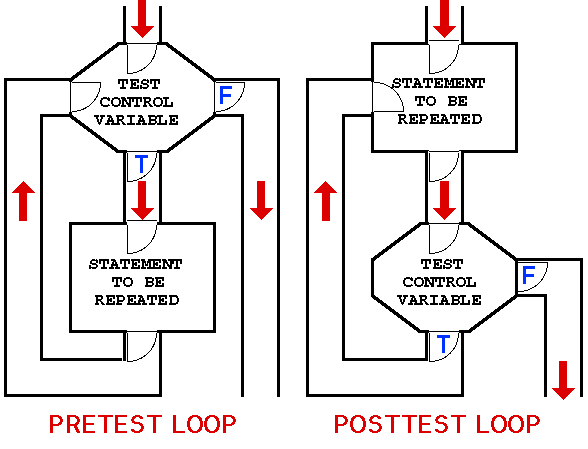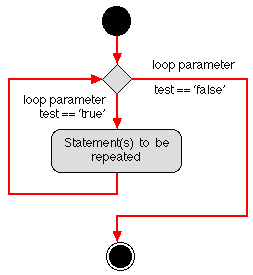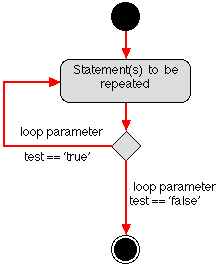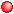# NOTE: This set of www pages is not the set of www pages for the curent version of COMP101. The pages are from a previous version that, at the request of students, I have kept on line.

## CONTENTS

 1. Repetition 1.1. pre-test and post-test loops 2. Loops in Java 3. For loops in Java (example) 4. Using the loop parameter (two examples) 5. Example problem - ASCII code output 5.1. Requirements 5.2. Analysis 5.3. Design ` ` 5.4. Implementation 5.5. Testing 6. Example problem - Arithmetic progression 6.1. Requirements 6.2. Analysis 6.3. Design 6.4. Implementation 6.5. Testing

The first three examples programmes illustrate fixed count pre-test loops using the "for" construct. The first example problem (ASCII character set) also includes a fixed count pre-test "for" loop. The second example problem (arithmetic progression) makes use of a variable count pre-test loop using a "for" construct.

## 1. REPETITION

 A repetition construct causes a group of one or more program statements to be invoked repeatedly until some end condition is met. The condition comprises a Boolean expression of some form. In this respect repetition constructs have three elements: Start expression Test expression Update expression ` ` We can identify two main forms of repetition: Fixed count loops - repeat a predefine number of times. Variable count loops (also sometimes referred to as conditional loops) - repeat an unspecified number of times (until some condition is met). To which we could add recursion (routine calls itself). We will confine the following discussion to fixed and variable count loops and briefly describe recursion at a later date.

### 1.1. pre-test and post-test loops

In addition both loops can be further classified according to whether they are pre-test or post-test loops. In the case of a pre-test loop the end condition is tested for prior to each repetition, while in the case of a post-test loop the end condition is tested for after each repetition. With respect to Java pre-test fixed and variable count loops are supported as well as post test fixed and variable count loops. Languages such as C and C++ support a similar set of loops, while languages such as Ada only support pre test loops.

` `

We can illustrate the distinction between pre-test and post-test loops by considering the flow of control associated with each. In the case of a pre-test loop we test before entering the "repeat" statement, thus if on the first attempt the test fails no repetitions will be made; in the case of a post-test loop the "repeat" statement is called at least once.

We can conceive of flow of control in terms of a floor plan comprising "rooms", "lobbies" and "corridors"; where "rooms" represent program statements, "lobbies" represent choice points, and "corridors" the connections between statements. We can then (in virtual terms) "run" round the floor plan simulating the flow of control. The floor plans given in Figure 1 thus simulate the flow of control that differentiates a pre-test loop from a post-test loop.

Generic activity diagrams for the pre- and post-test loop constructs are presented in Figure 2.Figure 1: Distinction between pre-test and post-test loops(a) ` `(b)

Figure 2: Generic activity diagrams for pre-test and post-test loops

## 2. LOOPS IN JAVA

 From the above we can therefore identify four basic types of loop: Fixed count pre-test loops. Fixed count post-test loops. Variable count pre-test loops. Variable count post-test loops. In Java (in common with languages such as C and C++) things are not so clear cut. Java supports three types of loop construct: ` ` For loops --- To define pre-test fixed or variable count loops. While loops --- To also define pre-test fixed and variable count loops. Do-while loops --- To define post-test fixed and variable count loops. Thus we have two mechanisms for constructing variable and fixed count pre-test loops; which to use and when is entirely up to the whim of the programmer!

## 3. FOR LOOPS IN JAVA

The general form of a for loop in Java is:

```for ( < startExpression > ;
< testExpression > ;
< updatExpression > ) {
< sequence of statements >
}
```

(Note the semi-colons.) This is a very concise (compared to languages such as Pascal and Ada), method of defining a loop (similar to that found in C and C++) that is sometimes criticised because of its lack of easy interpretation (there is always a trade off between conciseness and understandability). The counter-argument to this is that this form of loop definition is much more versatile than that available in (say) Ada. What ever the case, from the above:Start expression: Introduces one or more loop parameters (also referred to as the control variables or loop counters). These are introduced using an assignment expression which serves to assign a start value to the loop parameter. Where more than one loop parameter is introduced, each must be separated by a comma.Test expression: Defines the end condition for terminating the loop. Usually this a simple comparison expression. There can be more than one test expression in which case they must again be separated by commas.
` `Update expression: To avoid infinite repetition the loop parameter(s) must be updated on each repetition. This is usually done using an arithmetic expression. The most straight forward approach being to simply increment the loop parameter by one on each repetition. Again where there is more than one update expression they must be separated by commas.

Note: loop parameters are usually of some scalar numeric type. It is good practice to use parameters of type int or long. This is because real numbers have machine dependent precision associated with them, which may result in loops behaving in an unexpected manner as a result of inexact comparison of the loop parameter with some end condition.

In Table 1 a trivial Java program is presented, incorporating a loop, which outputs a sequence of 10 "smiley faces":

```(-:     (-:     (-:     (-:     (-:
(-:     (-:     (-:     (-:     (-:
```

Note that the start and end values for the loop are defined as constants and not as magic numbers. This is for sound software engineering reasons because the use of a name conveys much more meaning to a reader than a number.

 ```// SMILEY FACE APPLICATION CLASS // Frans Coenen // Tuesday 13 April 1999 // The University of Liverpool, UK class SmileyFace { // ------------------ METHODS ----------------------- /* Main method */ public static void main(String[] args) { int loopParameter; final int START_CONDITION = 0; final int END_CONDITION = 10; // For loop for (loopParameter = START_CONDITION;loopParameter < END_CONDITION; loopParameter++) System.out.print("(-:\t"); // End System.out.println("\n"); } } ```

Table 1: Java for loop example

In the example the loop parameter is declared before the loop construct and assigned to within the construct. We could have initialised the loop parameter entirely within the loop construct as follows:

```for (int loopParameter = START_CONDITION;loopParameter < END_CONDITION;
loopParameter++)
```

## 4. USING THE LOOP PARAMETER

In the example given in Table 1 the loop parameter serves no other purpose than to control the loop. In addition we can make use of the parameter as (say) part of an output statement, or as part of some simple arithmetic. Table 2 gives an example of the first, and Table 3 an example of the second. The code in Table 2 is used to simply output a sequence of numbers (the loop parameter):

```0 1 2 3 4 5 6 7 8 9
```

The code in Table 3 performs some arithmetic on the loop parameter so that a sequence of even numbers is produced:

```0 2 4 6 8 10 12 14 16 18
```
 ```// SEQUENCE NUMBERS CLASS // Frans Coenen // Tuesday 13 April 1999 // The University of Liverpool, UK class SequenceNumbers { // -------------- METHODS ------------------- /* Main method */ public static void main(String[] args) { final int START_CONDITION = 0; final int END_CONDITION = 10; // For loop for (int loopParam = START_CONDITION;loopParam < END_CONDITION; loopParam++) System.out.print(loopParam + " "); // End System.out.println("\n"); } } ```

Table 2 Sequence of numbers

 ```// SEQUENCE EVEN NUMBERS CLASS // Frans Coenen // Tuesday 13 April 1999 // The University of Liverpool, UK class SequenceEvenNumbers { // -------------- METHODS ------------------- /* Main method */ public static void main(String[] args) { final int START_CONDITION = 0; final int END_CONDITION = 10; // For loop for (int loopParam = START_CONDITION;loopParam < END_CONDITION; loopParam++) System.out.print(loopParam*2 + " "); // End System.out.println("\n"); } } ```

Table 3 Sequence of even numbers

The same result as that produced by the code in Table 3 can be achieved by rewriting the method as follows:

```public static void main(String[] args) {
final int START_CONDITION = 0;
final int END_CONDITION   = 19;

// For loop
for (int loopParam = START_CONDITION;loopParam < END_CONDITION;
loopParam = loopParam+2)
System.out.print(loopParam + " ");

// End
System.out.println("\n");
}
```

Created and maintained by Frans Coenen. Last updated 10 February 2015